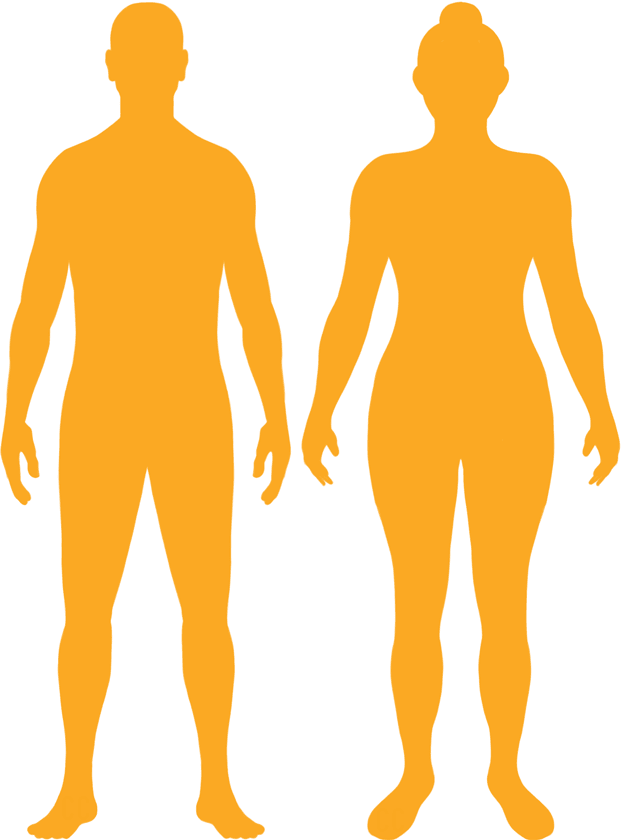# BMI Calculator

Result for 7'7" and 341 lbs.
Change Here.28.95

### Overweight BMI

At 7'7" and 341 Pounds your BMI is 28.95

### BMI Scale

 Under Normal Over Obese
-47 lbs
to reach a
normal BMI classification.
##### Normal BMI Ends:
294.5 lbs
+12 lbs
until you reach an obese BMI classification.
353.4 lbs

### BMI Grading Table2 for Height 7'7"

 Weight Weight Class Below 217.9 lbs Underweight 217.9 lbs - 294.5 lbs Normal Weight 294.5 lbs - 353.4 lbs Overweight 353.4 lbs and Above Obese

### Weight Loss

If you were seeking a normal BMI weight of 294.5lbs, and you lost two pounds a week4, then you could reach a normal BMI in
23.5 Weeks

### Overweight BMI Silhouette### Ideal Weight for a 7 foot 7 Male or Female2

The ideal weight range using the BMI for a male or female with a height of 7'7" is

### Between

217.9 lbs

and
294.5 lbs
How to Calculate BMI3 for 7'7" and 341 lbs
(7 x 12) + 7 = 91
(341 / (91 x 91)) x 703
= 28.948556937568
7 = foot | 7 = inch | 341 = weight lbs

### Conversion

• 7 foot 7 = 91 inches
• 7 foot 7 = 231.14cm
• 341 pounds = 24 stone 5 pounds
• 341 pounds = 154.67 kg
• 341 pounds = 154675 grams

### 7'7" and 341 lbs Summary

What is the BMI for a 7'7" and 341 lbs female? 28.95 BMI, Overweight.
What is the BMI for a 7'7" and 341 lbs male? 28.95 BMI, Overweight.
What is the ideal weight for a 7'7" female? Between: 217.9lbs and 294.5lbs
What is the ideal weight for a 7'7" male? Between: 217.9lbs and 294.5lbs
If I am 7ft 7in and weigh 341 lbs, is that a good weight for my height? Under the BMI classification, 341 lbs is classed as being Overweight.